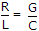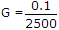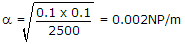# Electronics and Communication Engineering - Exam Questions Papers - Discussion

Discussion Forum : Exam Questions Papers - Exam Paper 1 (Q.No. 2)
2.
A transmission line has a characteristic impedance of 50 Ω and a resistance of 0.1 Ω/m, if the line is distortion less, the attenuation constant (in Np/m) is
500
5
0.014
0.002
Explanation:

Distortion lessa = RG and Z0 = RG.

Discussion:
23 comments Page 1 of 3.

Raey said:   4 years ago
Thanks for explaining @Jacb.

Ravi said:   4 years ago
Thanks @Jacb.

Charan said:   5 years ago
It's clear now, Thanks @Jacb.

Jacb said:   6 years ago
z0=√(R/G) , equate to G: G=R/(z0)^2.
atten const = √(R*G), subs. G :
atten const = √(R*(R/(z0)^2)).
atten const = √(0.1 * ( 0.1/ (50)^2)).

Hope that helps.
(1)

NIDHEESH NELSON said:   6 years ago
Can any one explain this?

Mahesh said:   6 years ago
Can anyone explain me briefly?

Sachin kumar said:   6 years ago
How 0.1/2*50=0.1/2500 can anyone explain?

Shweta said:   6 years ago
How it is?

Zo= √ RG.
It is Zo= √( R/G).

Ashwin said:   7 years ago
We know that in distortion less medium R/L=G/C, so alpha= R.sqrt(C/L) and Zo= sqrt(L/C), so alpha= R/Zo.

Sonal said:   7 years ago
R = α * z0. Compute by this formula.